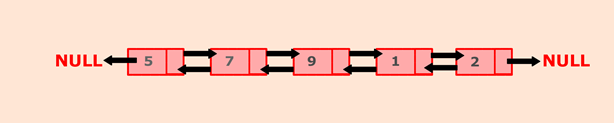Q:

# Java program to find the maximum and minimum value node from a doubly linked list

belongs to collection: Java Doubly Linked List Programs

In this program, we will create a doubly linked list then, iterate through the list to find out the minimum and maximum node.We will maintain two variables min and max. Min will hold the minimum value node, and max will hold the maximum value node. In above example, 1 will be the minimum value node and 9 will be the maximum value node.

### Algorithm

• Define a Node class which represents a node in the list. It will have three properties: data, previous which will point to the previous node and next which will point to the next node.
• Define another class for creating the doubly linked list, and it has two nodes: head and tail. Initially, head and tail will point to null.
• minimumNode() will prints out minimum value node:
• Define variable min and initialize with head's data.
• Current will point to head.
• Iterate through the list by comparing each node's data with min.
• If min > current's data then min will hold current's data.
• At the end of the list, variable min will hold the minimum value node.
• Print the min value.

a. maximumNode() will prints out maximum value node:

• Define variable max and initialize with head's data.
• Current will point to head.
• Iterate through the list by comparing each node's data with max.
• If max < current's data then max will hold current?s data.
• At the end of the list, variable max will hold the maximum value node.
• Print the max value.

### Program:

``````public class MinMax {

//Represent a node of the doubly linked list

class Node{
int data;
Node previous;
Node next;

public Node(int data) {
this.data = data;
}
}

//Create a new node
Node newNode = new Node(data);

//If list is empty
//Both head and tail will point to newNode
//head's previous will point to null
//tail's next will point to null, as it is the last node of the list
tail.next = null;
}
else {
//newNode will be added after tail such that tail's next will point to newNode
tail.next = newNode;
//newNode's previous will point to tail
newNode.previous = tail;
//newNode will become new tail
tail = newNode;
//As it is last node, tail's next will point to null
tail.next = null;
}
}

//MinimumNode() will find out minimum value node in the list
public int minimumNode() {
//Node current will point to head
int min;

//Checks if list is empty
System.out.println("List is empty");
return 0;
}
else {
//Initially, min will store the value of head's data
while(current != null) {
//If the value of min is greater than the current's data

//Then, replace the value of min with current node's data

if(min > current.data)
min = current.data;
current = current.next;
}
}
return min;
}

//MaximumNode() will find out maximum value node in the list
public int maximumNode() {
//Node current will point to head
int max;

//Checks if list is empty
System.out.println("List is empty");
return 0;
}
else {
//Initially, max will store the value of head's data
//If value of max is lesser than current's data
//Then, replace value of max with current node's data
while(current != null) {
if(current.data > max)
max = current.data;
current = current.next;
}
}
return max;
}

public static void main(String[] args) {

MinMax dList = new MinMax();

//Prints the minimum value node in the list
System.out.println("Minimum value node in the list: "+ dList.minimumNode());
//Prints the maximum value node in the list
System.out.println("Maximum value node in the list: "+ dList.maximumNode());
}
}  ``````

Output:

```Minimum value node in the list: 1
Maximum value node in the list: 9```